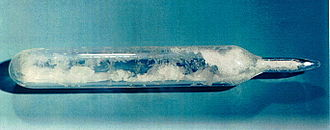# Homework #3

$$\newcommand{\vecs}{\overset { \rightharpoonup} {\mathbf{#1}} }$$ $$\newcommand{\vecd}{\overset{-\!-\!\rightharpoonup}{\vphantom{a}\smash {#1}}}$$$$\newcommand{\id}{\mathrm{id}}$$ $$\newcommand{\Span}{\mathrm{span}}$$ $$\newcommand{\kernel}{\mathrm{null}\,}$$ $$\newcommand{\range}{\mathrm{range}\,}$$ $$\newcommand{\RealPart}{\mathrm{Re}}$$ $$\newcommand{\ImaginaryPart}{\mathrm{Im}}$$ $$\newcommand{\Argument}{\mathrm{Arg}}$$ $$\newcommand{\norm}{\| #1 \|}$$ $$\newcommand{\inner}{\langle #1, #2 \rangle}$$ $$\newcommand{\Span}{\mathrm{span}}$$ $$\newcommand{\id}{\mathrm{id}}$$ $$\newcommand{\Span}{\mathrm{span}}$$ $$\newcommand{\kernel}{\mathrm{null}\,}$$ $$\newcommand{\range}{\mathrm{range}\,}$$ $$\newcommand{\RealPart}{\mathrm{Re}}$$ $$\newcommand{\ImaginaryPart}{\mathrm{Im}}$$ $$\newcommand{\Argument}{\mathrm{Arg}}$$ $$\newcommand{\norm}{\| #1 \|}$$ $$\newcommand{\inner}{\langle #1, #2 \rangle}$$ $$\newcommand{\Span}{\mathrm{span}}$$

## 3.1

Write the products of these acid-base reactions. Balance the complete reaction.

1. $$HF_{(aq)} + KOH_{(aq)} \rightarrow$$
2. $$H_2SO_{4\;(aq)} + Ca(OH)_{2\;(aq)} \rightarrow$$

## 3.2

Balance the following redox reaction in basic conditions with state symbols.

$H_2O_{(l)} + Na_{(s)} \rightarrow Na^+_{(aq)} + H_{2\;(g)}$

## 3.3

In a lab experiment, a 20.00 ml $$HNO_2$$ solution was titrated with $$0.1234\; M$$ sodium hydroxide solution, and the equivalence point was reached when 43.21 ml of this basic solution was added. What is the original molarity of the $$HNO_2$$ solution?

## 3.4

What is the required mL of 1.25 M $$HBr$$ necessary to completely neutralize a 175 mL sample of 2.38 M $$Mg(OH)_2$$?

## 3.5

A rubber duck is initially filled with 100 mL air at $$2 ^oC$$ in the bathroom and then set in the sun to be heated. The final temperature increases to $$10 ^oC$$ at constant pressure.What is the final condition of the rubber duck? Does it shrink or expand? What is its final volume?

## 3.6

A commercial balloon is filled with 3000 L helium gas at $$30^oC$$. If the pressure is 1 atm, what is the mass of helium gas? Why does the balloon float?

## 3.7

Uranium hexafluoride ($$UF_6$$) is a compound used in the uranium enrichment process that produces fuel for nuclear reactors and nuclear weapons. It forms solid grey crystals at standard temperature and pressure and boils at 56°C.Figure: Uranium hexafluoride crystals sealed in an ampoule

The density of uranium hexafluoride is 12.6 g/L at 745 torr. What is the temperature in °C under these condition?

## 3.8

A constant-volume container has 2.00 moles gas sample at 25 °C and the gas sample exerts a pressure of 450 torr. If 2.00 moles more gas is added in the container and the temperature is increased to 50 °C, what is the pressure in atm?

Homework #3 is shared under a not declared license and was authored, remixed, and/or curated by LibreTexts.